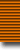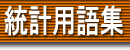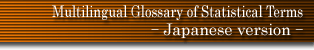### 用語検索

and or
検索単位 単語 フレーズ 完全一致 〜で始まる 〜を含む 〜で終わる 日本語 英語 ドイツ語 フランス語
 日本語 英語 ドイツ語 フランス語
 factor factor analysis factor antithesis factor loading factor matrix factor pattern factor reversal test factor rotation factor score factorial cumulant factorial cumulant generating function factorial distribution factorial experiment factorial moment factorial moment generating function factorial multinomial distribution factorial sum failure rate fair game fast Fourier transform Fatou's lemma F-distribution feature selection Fellegi's method Fermi-Dirac statistics Ferreri distribution fertility gradient fertility rate FFT fiducial distribution fiducial inference fiducial limits fiducial probability Fieller-Hartley-Pearson measure of association Fieller's theorem filter finite arc sine distribution finite Markov chain finite multiplier finite population finite population correction finite sampling correction first digit distribution first limit theorem first passage time first return time first stage unit Fisher information Fisher model Fisher-Behrens Test Fisher-Hsu-Roy distribution Fisher-Irwin test Fisher's (spherical Normal) distribution Fisher's B-distribution Fisher's distribution Fisher's transformation (of the correlation coefficient) Fisher-Yates test five-point assay fixed base index fixed effects (constants) model fixed sample fixed variate flexibility curve fluctuation Fokker-Planck equation folded contingency table folded distributions folded median folded Normal distribution folding follow-up force of mortality forecasting forward equations Foster's criteria fourfold table Fourier analysis Fourier transform Fréchet distribution Fréchet's inequalities fractal fractile fractile graphical analysis fraction defective fractional replication frame freehand method Freeman-Tukey transformation frequency frequency curve frequency distribution frequency domain frequency function frequency moment frequency polygon frequency response function frequency surface frequency table frequency theory of probability frequentist Freund-Ansari test Friedman's test F-test full information method functional central limit theorem functional relationship fundamental probability set fundamental random process Furry process fuzzy set theory